# fluid.pptx

31 de May de 2023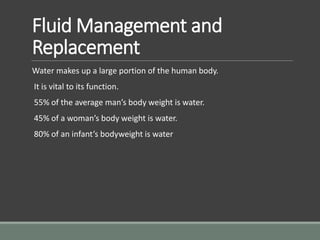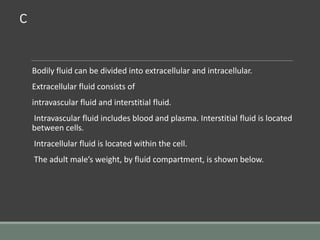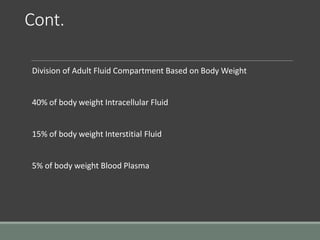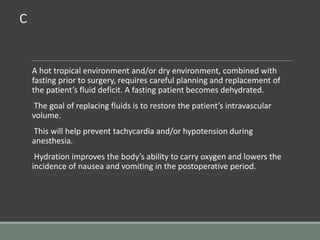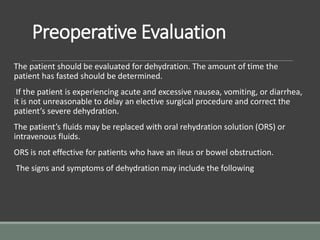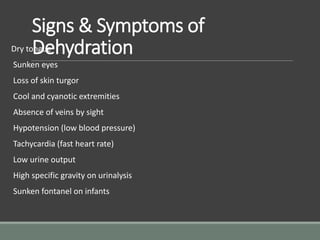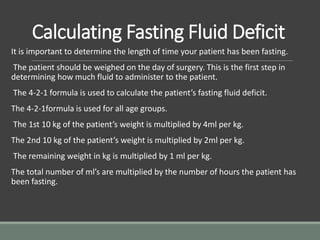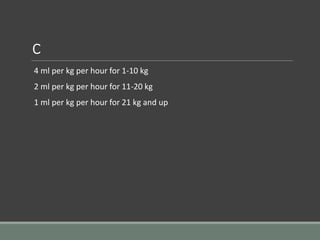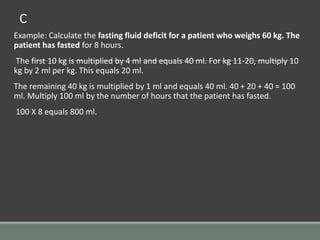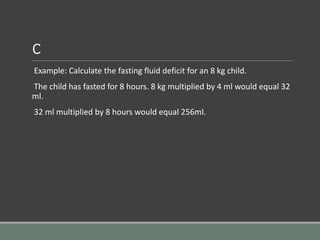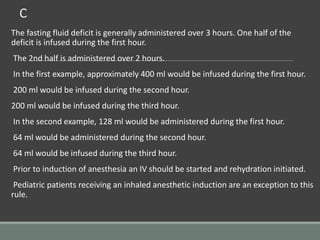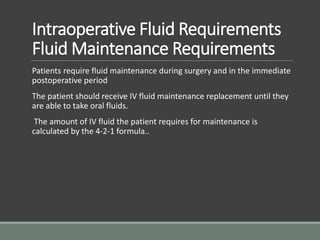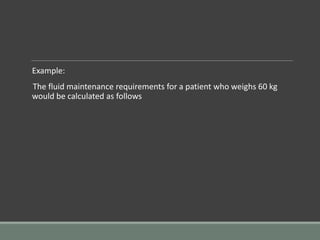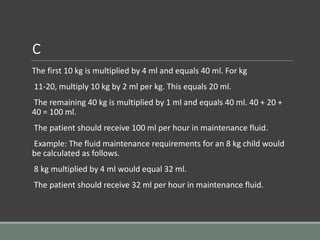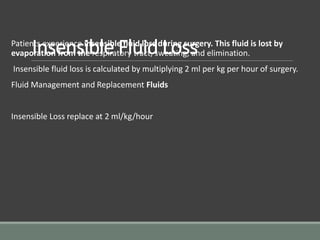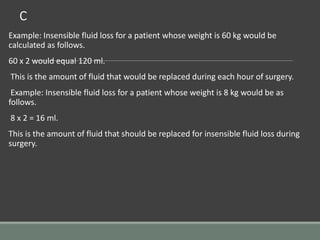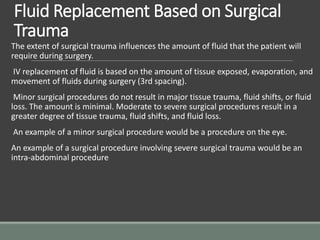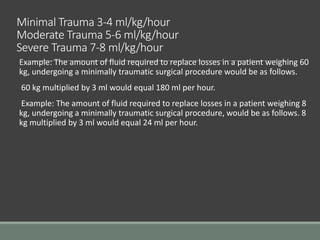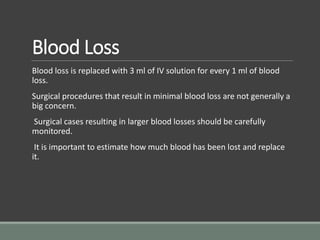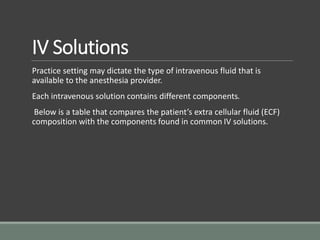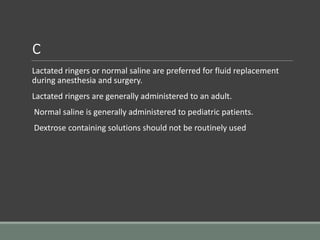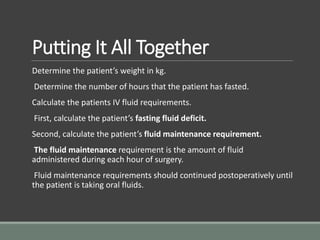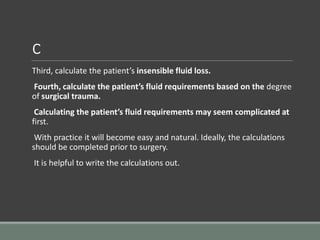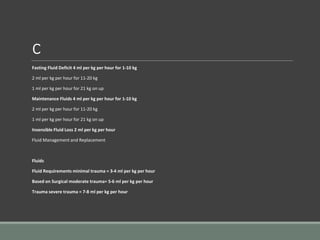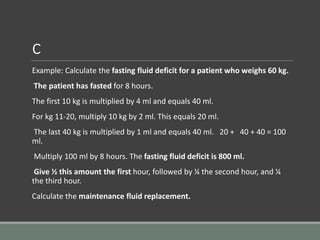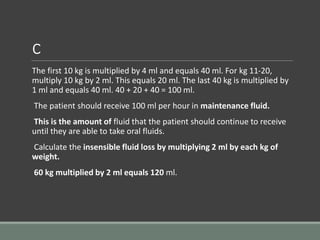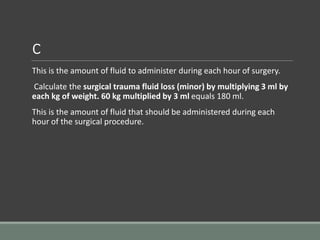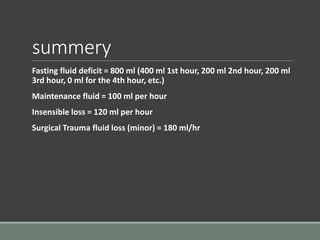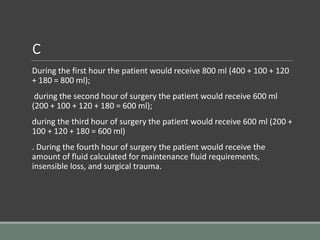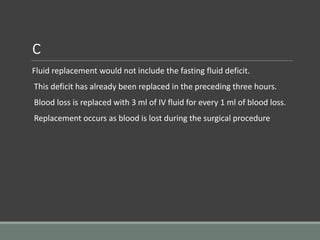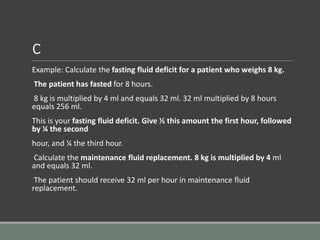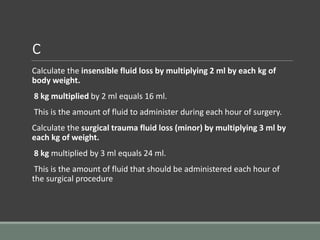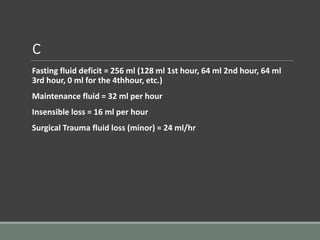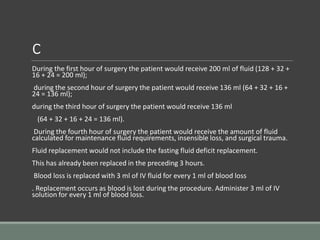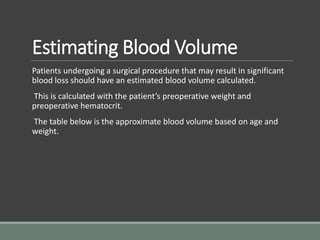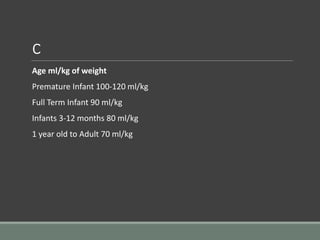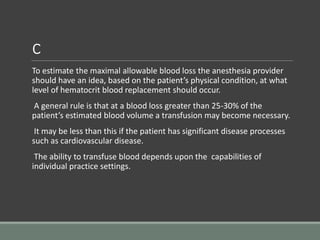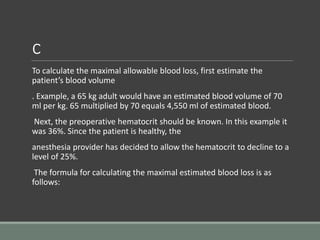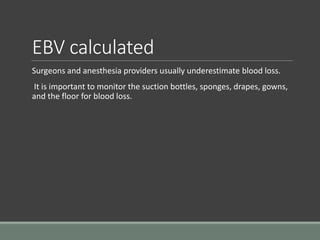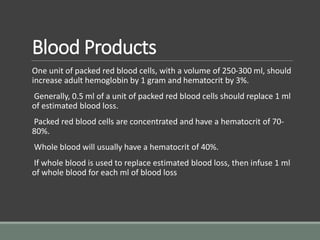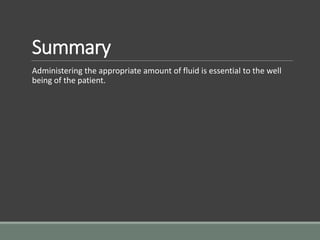1 de 45

### fluid.pptx

• 1. Transfusion By K/Mariam Tamrat MSc Anaesthesiology 2015
• 2. Course Objectives BY THE END OF THE COURSE THE STUDENTS WILL BE ABLE TO: DESCRIBE THE BODY FLUID COMPOSITION AND COMPARTMENTS DIAGNOSE FLUID, ELECTROLYTE, AND ACID-BASE ABNORMALITIES, EXPLAIN THE PRINCIPLES OF FLUID THERAPY MANAGE FLUID, ELECTROLYTE, AND ACID-BASE ABNORMALITIES
• 3. c Fluid Management andReplacement
• 4. Fluid Management and Replacement Water makes up a large portion of the human body. It is vital to its function. 55% of the average man’s body weight is water. 45% of a woman’s body weight is water. 80% of an infant’s bodyweight is water
• 5. c Bodily fluid can be divided into extracellular and intracellular. Extracellular fluid consists of intravascular fluid and interstitial fluid. Intravascular fluid includes blood and plasma. Interstitial fluid is located between cells. Intracellular fluid is located within the cell. The adult male’s weight, by fluid compartment, is shown below.
• 6. Cont. Division of Adult Fluid Compartment Based on Body Weight 40% of body weight Intracellular Fluid 15% of body weight Interstitial Fluid 5% of body weight Blood Plasma
• 7. c A hot tropical environment and/or dry environment, combined with fasting prior to surgery, requires careful planning and replacement of the patient’s fluid deficit. A fasting patient becomes dehydrated. The goal of replacing fluids is to restore the patient’s intravascular volume. This will help prevent tachycardia and/or hypotension during anesthesia. Hydration improves the body’s ability to carry oxygen and lowers the incidence of nausea and vomiting in the postoperative period.
• 8. Preoperative Evaluation The patient should be evaluated for dehydration. The amount of time the patient has fasted should be determined. If the patient is experiencing acute and excessive nausea, vomiting, or diarrhea, it is not unreasonable to delay an elective surgical procedure and correct the patient’s severe dehydration. The patient’s fluids may be replaced with oral rehydration solution (ORS) or intravenous fluids. ORS is not effective for patients who have an ileus or bowel obstruction. The signs and symptoms of dehydration may include the following
• 9. Signs & Symptoms of Dehydration Dry tongue Sunken eyes Loss of skin turgor Cool and cyanotic extremities Absence of veins by sight Hypotension (low blood pressure) Tachycardia (fast heart rate) Low urine output High specific gravity on urinalysis Sunken fontanel on infants
• 10. Calculating Fasting Fluid Deficit It is important to determine the length of time your patient has been fasting. The patient should be weighed on the day of surgery. This is the first step in determining how much fluid to administer to the patient. The 4-2-1 formula is used to calculate the patient’s fasting fluid deficit. The 4-2-1formula is used for all age groups. The 1st 10 kg of the patient’s weight is multiplied by 4ml per kg. The 2nd 10 kg of the patient’s weight is multiplied by 2ml per kg. The remaining weight in kg is multiplied by 1 ml per kg. The total number of ml’s are multiplied by the number of hours the patient has been fasting.
• 11. c 4 ml per kg per hour for 1-10 kg 2 ml per kg per hour for 11-20 kg 1 ml per kg per hour for 21 kg and up
• 12. c Example: Calculate the fasting fluid deficit for a patient who weighs 60 kg. The patient has fasted for 8 hours. The first 10 kg is multiplied by 4 ml and equals 40 ml. For kg 11-20, multiply 10 kg by 2 ml per kg. This equals 20 ml. The remaining 40 kg is multiplied by 1 ml and equals 40 ml. 40 + 20 + 40 = 100 ml. Multiply 100 ml by the number of hours that the patient has fasted. 100 X 8 equals 800 ml.
• 13. c Example: Calculate the fasting fluid deficit for an 8 kg child. The child has fasted for 8 hours. 8 kg multiplied by 4 ml would equal 32 ml. 32 ml multiplied by 8 hours would equal 256ml.
• 14. c The fasting fluid deficit is generally administered over 3 hours. One half of the deficit is infused during the first hour. The 2nd half is administered over 2 hours. In the first example, approximately 400 ml would be infused during the first hour. 200 ml would be infused during the second hour. 200 ml would be infused during the third hour. In the second example, 128 ml would be administered during the first hour. 64 ml would be administered during the second hour. 64 ml would be infused during the third hour. Prior to induction of anesthesia an IV should be started and rehydration initiated. Pediatric patients receiving an inhaled anesthetic induction are an exception to this rule.
• 15. Intraoperative Fluid Requirements Fluid Maintenance Requirements Patients require fluid maintenance during surgery and in the immediate postoperative period The patient should receive IV fluid maintenance replacement until they are able to take oral fluids. The amount of IV fluid the patient requires for maintenance is calculated by the 4-2-1 formula..
• 16. Example: The fluid maintenance requirements for a patient who weighs 60 kg would be calculated as follows
• 17. c The first 10 kg is multiplied by 4 ml and equals 40 ml. For kg 11-20, multiply 10 kg by 2 ml per kg. This equals 20 ml. The remaining 40 kg is multiplied by 1 ml and equals 40 ml. 40 + 20 + 40 = 100 ml. The patient should receive 100 ml per hour in maintenance fluid. Example: The fluid maintenance requirements for an 8 kg child would be calculated as follows. 8 kg multiplied by 4 ml would equal 32 ml. The patient should receive 32 ml per hour in maintenance fluid.
• 18. Insensible Fluid Loss Patients experience insensible fluid loss during surgery. This fluid is lost by evaporation from the respiratory tract, sweating, and elimination. Insensible fluid loss is calculated by multiplying 2 ml per kg per hour of surgery. Fluid Management and Replacement Fluids Insensible Loss replace at 2 ml/kg/hour
• 19. c Example: Insensible fluid loss for a patient whose weight is 60 kg would be calculated as follows. 60 x 2 would equal 120 ml. This is the amount of fluid that would be replaced during each hour of surgery. Example: Insensible fluid loss for a patient whose weight is 8 kg would be as follows. 8 x 2 = 16 ml. This is the amount of fluid that should be replaced for insensible fluid loss during surgery.
• 20. Fluid Replacement Based on Surgical Trauma The extent of surgical trauma influences the amount of fluid that the patient will require during surgery. IV replacement of fluid is based on the amount of tissue exposed, evaporation, and movement of fluids during surgery (3rd spacing). Minor surgical procedures do not result in major tissue trauma, fluid shifts, or fluid loss. The amount is minimal. Moderate to severe surgical procedures result in a greater degree of tissue trauma, fluid shifts, and fluid loss. An example of a minor surgical procedure would be a procedure on the eye. An example of a surgical procedure involving severe surgical trauma would be an intra-abdominal procedure
• 21. Minimal Trauma 3-4 ml/kg/hour Moderate Trauma 5-6 ml/kg/hour Severe Trauma 7-8 ml/kg/hour Example: The amount of fluid required to replace losses in a patient weighing 60 kg, undergoing a minimally traumatic surgical procedure would be as follows. 60 kg multiplied by 3 ml would equal 180 ml per hour. Example: The amount of fluid required to replace losses in a patient weighing 8 kg, undergoing a minimally traumatic surgical procedure, would be as follows. 8 kg multiplied by 3 ml would equal 24 ml per hour.
• 22. Blood Loss Blood loss is replaced with 3 ml of IV solution for every 1 ml of blood loss. Surgical procedures that result in minimal blood loss are not generally a big concern. Surgical cases resulting in larger blood losses should be carefully monitored. It is important to estimate how much blood has been lost and replace it.
• 23. IV Solutions Practice setting may dictate the type of intravenous fluid that is available to the anesthesia provider. Each intravenous solution contains different components. Below is a table that compares the patient’s extra cellular fluid (ECF) composition with the components found in common IV solutions.
• 24. c Lactated ringers or normal saline are preferred for fluid replacement during anesthesia and surgery. Lactated ringers are generally administered to an adult. Normal saline is generally administered to pediatric patients. Dextrose containing solutions should not be routinely used
• 25. Putting It All Together Determine the patient’s weight in kg. Determine the number of hours that the patient has fasted. Calculate the patients IV fluid requirements. First, calculate the patient’s fasting fluid deficit. Second, calculate the patient’s fluid maintenance requirement. The fluid maintenance requirement is the amount of fluid administered during each hour of surgery. Fluid maintenance requirements should continued postoperatively until the patient is taking oral fluids.
• 26. c Third, calculate the patient’s insensible fluid loss. Fourth, calculate the patient’s fluid requirements based on the degree of surgical trauma. Calculating the patient’s fluid requirements may seem complicated at first. With practice it will become easy and natural. Ideally, the calculations should be completed prior to surgery. It is helpful to write the calculations out.
• 27. c Fasting Fluid Deficit 4 ml per kg per hour for 1-10 kg 2 ml per kg per hour for 11-20 kg 1 ml per kg per hour for 21 kg on up Maintenance Fluids 4 ml per kg per hour for 1-10 kg 2 ml per kg per hour for 11-20 kg 1 ml per kg per hour for 21 kg on up Insensible Fluid Loss 2 ml per kg per hour Fluid Management and Replacement Fluids Fluid Requirements minimal trauma = 3-4 ml per kg per hour Based on Surgical moderate trauma= 5-6 ml per kg per hour Trauma severe trauma = 7-8 ml per kg per hour
• 28. c Example: Calculate the fasting fluid deficit for a patient who weighs 60 kg. The patient has fasted for 8 hours. The first 10 kg is multiplied by 4 ml and equals 40 ml. For kg 11-20, multiply 10 kg by 2 ml. This equals 20 ml. The last 40 kg is multiplied by 1 ml and equals 40 ml. 20 + 40 + 40 = 100 ml. Multiply 100 ml by 8 hours. The fasting fluid deficit is 800 ml. Give ½ this amount the first hour, followed by ¼ the second hour, and ¼ the third hour. Calculate the maintenance fluid replacement.
• 29. c The first 10 kg is multiplied by 4 ml and equals 40 ml. For kg 11-20, multiply 10 kg by 2 ml. This equals 20 ml. The last 40 kg is multiplied by 1 ml and equals 40 ml. 40 + 20 + 40 = 100 ml. The patient should receive 100 ml per hour in maintenance fluid. This is the amount of fluid that the patient should continue to receive until they are able to take oral fluids. Calculate the insensible fluid loss by multiplying 2 ml by each kg of weight. 60 kg multiplied by 2 ml equals 120 ml.
• 30. c This is the amount of fluid to administer during each hour of surgery. Calculate the surgical trauma fluid loss (minor) by multiplying 3 ml by each kg of weight. 60 kg multiplied by 3 ml equals 180 ml. This is the amount of fluid that should be administered during each hour of the surgical procedure.
• 31. summery Fasting fluid deficit = 800 ml (400 ml 1st hour, 200 ml 2nd hour, 200 ml 3rd hour, 0 ml for the 4th hour, etc.) Maintenance fluid = 100 ml per hour Insensible loss = 120 ml per hour Surgical Trauma fluid loss (minor) = 180 ml/hr
• 32. c During the first hour the patient would receive 800 ml (400 + 100 + 120 + 180 = 800 ml); during the second hour of surgery the patient would receive 600 ml (200 + 100 + 120 + 180 = 600 ml); during the third hour of surgery the patient would receive 600 ml (200 + 100 + 120 + 180 = 600 ml) . During the fourth hour of surgery the patient would receive the amount of fluid calculated for maintenance fluid requirements, insensible loss, and surgical trauma.
• 33. c Fluid replacement would not include the fasting fluid deficit. This deficit has already been replaced in the preceding three hours. Blood loss is replaced with 3 ml of IV fluid for every 1 ml of blood loss. Replacement occurs as blood is lost during the surgical procedure
• 34. c Example: Calculate the fasting fluid deficit for a patient who weighs 8 kg. The patient has fasted for 8 hours. 8 kg is multiplied by 4 ml and equals 32 ml. 32 ml multiplied by 8 hours equals 256 ml. This is your fasting fluid deficit. Give ½ this amount the first hour, followed by ¼ the second hour, and ¼ the third hour. Calculate the maintenance fluid replacement. 8 kg is multiplied by 4 ml and equals 32 ml. The patient should receive 32 ml per hour in maintenance fluid replacement.
• 35. c Calculate the insensible fluid loss by multiplying 2 ml by each kg of body weight. 8 kg multiplied by 2 ml equals 16 ml. This is the amount of fluid to administer during each hour of surgery. Calculate the surgical trauma fluid loss (minor) by multiplying 3 ml by each kg of weight. 8 kg multiplied by 3 ml equals 24 ml. This is the amount of fluid that should be administered each hour of the surgical procedure
• 36. c Fasting fluid deficit = 256 ml (128 ml 1st hour, 64 ml 2nd hour, 64 ml 3rd hour, 0 ml for the 4thhour, etc.) Maintenance fluid = 32 ml per hour Insensible loss = 16 ml per hour Surgical Trauma fluid loss (minor) = 24 ml/hr
• 37. c During the first hour of surgery the patient would receive 200 ml of fluid (128 + 32 + 16 + 24 = 200 ml); during the second hour of surgery the patient would receive 136 ml (64 + 32 + 16 + 24 = 136 ml); during the third hour of surgery the patient would receive 136 ml (64 + 32 + 16 + 24 = 136 ml). During the fourth hour of surgery the patient would receive the amount of fluid calculated for maintenance fluid requirements, insensible loss, and surgical trauma. Fluid replacement would not include the fasting fluid deficit replacement. This has already been replaced in the preceding 3 hours. Blood loss is replaced with 3 ml of IV fluid for every 1 ml of blood loss . Replacement occurs as blood is lost during the procedure. Administer 3 ml of IV solution for every 1 ml of blood loss.
• 38. NB. Drawing a simple grid can help keep track of fluid replacement. 1st Hour 2nd Hour 3rd Hour 4th Hour Fluid Deficit Maintenance Fluid Surgical Trauma Blood Loss Total
• 39. Estimating Blood Volume Patients undergoing a surgical procedure that may result in significant blood loss should have an estimated blood volume calculated. This is calculated with the patient’s preoperative weight and preoperative hematocrit. The table below is the approximate blood volume based on age and weight.
• 40. c Age ml/kg of weight Premature Infant 100-120 ml/kg Full Term Infant 90 ml/kg Infants 3-12 months 80 ml/kg 1 year old to Adult 70 ml/kg
• 41. c To estimate the maximal allowable blood loss the anesthesia provider should have an idea, based on the patient’s physical condition, at what level of hematocrit blood replacement should occur. A general rule is that at a blood loss greater than 25-30% of the patient’s estimated blood volume a transfusion may become necessary. It may be less than this if the patient has significant disease processes such as cardiovascular disease. The ability to transfuse blood depends upon the capabilities of individual practice settings.
• 42. c To calculate the maximal allowable blood loss, first estimate the patient’s blood volume . Example, a 65 kg adult would have an estimated blood volume of 70 ml per kg. 65 multiplied by 70 equals 4,550 ml of estimated blood. Next, the preoperative hematocrit should be known. In this example it was 36%. Since the patient is healthy, the anesthesia provider has decided to allow the hematocrit to decline to a level of 25%. The formula for calculating the maximal estimated blood loss is as follows:
• 43. EBV calculated Surgeons and anesthesia providers usually underestimate blood loss. It is important to monitor the suction bottles, sponges, drapes, gowns, and the floor for blood loss.
• 44. Blood Products One unit of packed red blood cells, with a volume of 250-300 ml, should increase adult hemoglobin by 1 gram and hematocrit by 3%. Generally, 0.5 ml of a unit of packed red blood cells should replace 1 ml of estimated blood loss. Packed red blood cells are concentrated and have a hematocrit of 70- 80%. Whole blood will usually have a hematocrit of 40%. If whole blood is used to replace estimated blood loss, then infuse 1 ml of whole blood for each ml of blood loss
• 45. Summary Administering the appropriate amount of fluid is essential to the well being of the patient.